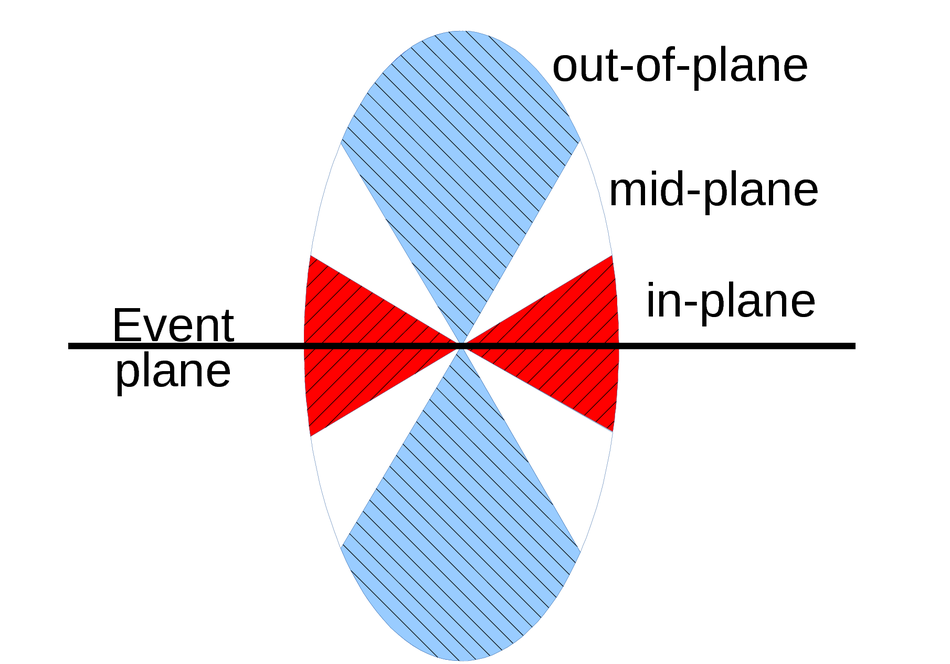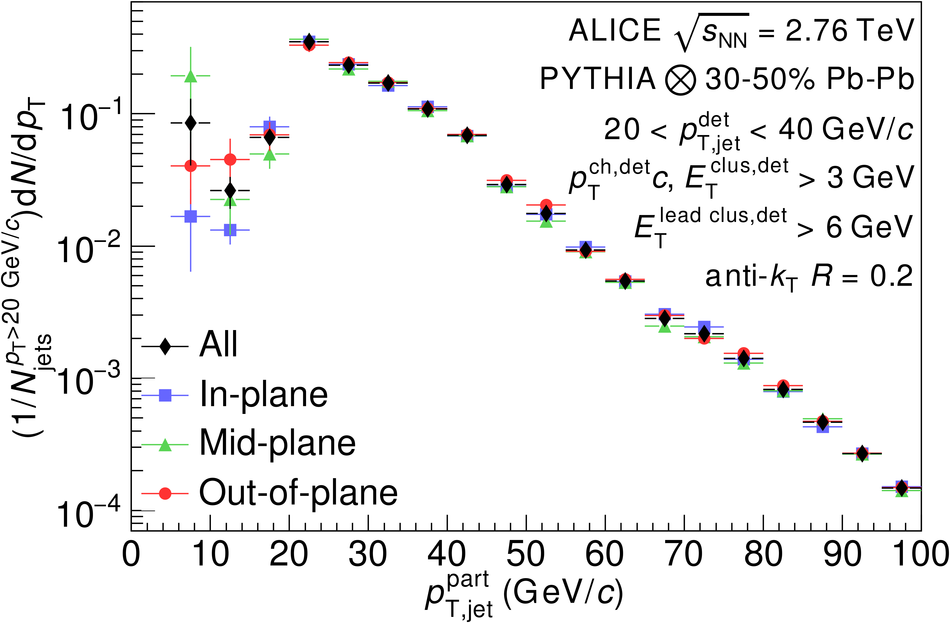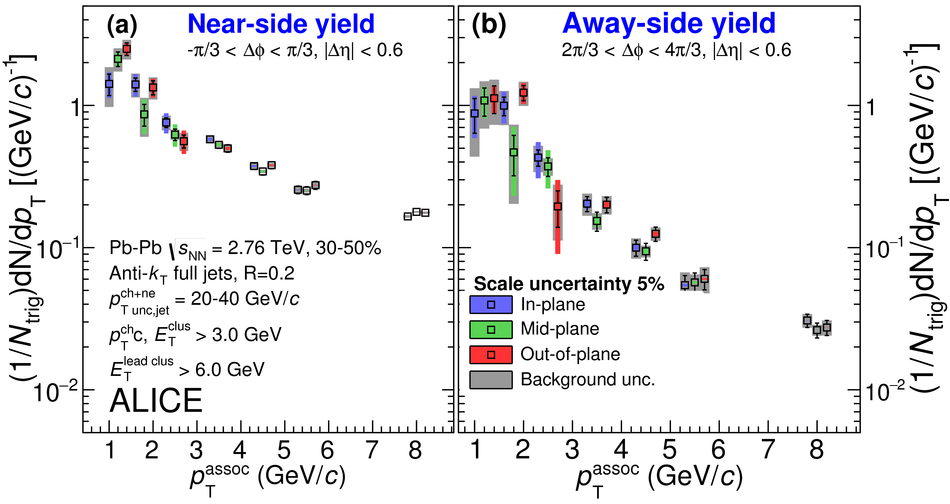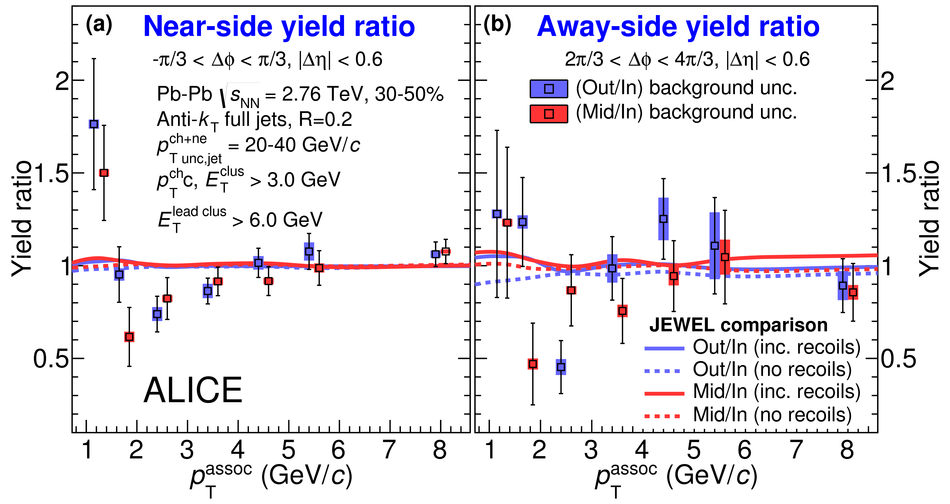# Jet-hadron correlations measured relative to the second order event plane in Pb-Pb collisions at $\sqrt{s_{\rm{NN}}}$ = 2.76 TeV

The Quark Gluon Plasma (QGP) produced in ultra relativistic heavy-ion collisions at the Large Hadron Collider (LHC) can be studied by measuring the modifications of jets formed by hard scattered partons which interact with the medium. We studied these modifications via angular correlations of jets with charged hadrons for jets with momenta 20 <~ $p_{\rm{T}}^{\rm{jet}}$ <~ 40 GeV/$c$ as a function of the associated particle momentum. The reaction plane fit (RPF) method is used in this analysis to remove the flow modulated background. The analysis of angular correlations for different orientations of the jet relative to the second order event plane allows for the study of the path length dependence of medium modifications to jets. We present the dependence of azimuthal angular correlations of charged hadrons with respect to the angle of the axis of a reconstructed jet relative to the event plane in Pb-Pb collisions at $\sqrt{s_{\rm{NN}}}$ = 2.76 TeV. The dependence of particle yields associated with jets on the angle of the jet with respect to the event plane is presented. Correlations at different angles relative to the event plane are compared through ratios and differences of the yield. No dependence of the results on the angle of the jet with respect to the event plane is observed within uncertainties, which is consistent with no significant path length dependence of the medium modifications for this observable.

Figures

## Figure 1

 Jet-hadron correlations are measured for jets in three regions relative to the $n = 2$ event plane, which is transverse to the direction of the beams. These regions include in-plane ($| \psi-\phi_{\mathrm {jet}}|< \pi/6$) shown in red, mid-plane ($\pi/6< |\psi-\phi_{\mathrm {jet}}|< \pi/3$) shown in white, and out-of-plane ($|\psi-\phi_{\mathrm {jet}}|>\pi/3$) shown in blue## Figure 2

 The generator level jet probability distribution corresponding to jets measured with \pt between 20-40 \GeV for PYTHIA events embedded in 30--50\% central \PbPb collisions. Generator level jets are required to have $\pt>5$ \GeV. The distribution is measuredfor each angle relative to the event plane, as well as the sum of all angles.## Figure 3

 The signal plus background region, $|\Delta\eta|< 0.6$ (green points), the region which is background-dominated on the \ns, $0.8< |\Delta\eta|< 1.2$ (black points), and the RPF fit to $|\Delta\phi|< \pi/2$ (blue band) to the region which is background-dominated on the \ns for \pttrigrange{20}{40} jets correlated with \ptassocrange{1.5}{2.0} hadrons from 30-50\% centrality collisions on the top panel. The middle panel shows the quality of the RPF fit to the region which is background-dominated on the \ns, (data$-$fit)/fit. On the bottom panel are the RPF corrected correlation functions, with the uncertainty from the background fit (red band), and the correlated uncertainty (green band)## Figure 4

 The (a) near-side and (b) away-side yield vs \ptassoc for \pttrigrange{20}{40} full jets of 30-50\% centrality in \PbPb collisions. The wider band corresponds to the background uncertainty, which is non-trivially correlated point-to-point~. The narrower bands are the systematic uncertainties coming from the shape uncertainty of the acceptance correction. There is an additional 5\% global scale uncertainty. Points are displaced for visibility.## Figure 5

 The (a) near-side and (b) away-side yield differences vs \ptassoc for \pttrigrange{20}{40} full jets of 30-50\% centrality in \PbPb collisions. The narrower band corresponds to the background uncertainty, which is non-trivially correlated point-to-point~. The wider bands are the systematic uncertainties coming from the shape uncertainty of the acceptance correction. There is an additional 5\% global scale uncertainty. Points are displaced for visibility. Data are compared to calculations from JEWEL~ with and without recoil particles## Figure 6

 The (a) near-side and (b) away-side yield ratios vs \ptassoc for \pttrigrange{20}{40} full jets of 30-50\% centrality in \PbPb collisions. The bands correspond to the background uncertainty, which is non-trivially correlated point-to-point~. The systematic uncertainties coming from the shape uncertainty of the acceptance correction cancel out for the ratios. Points are displaced for visibility. Data are compared to calculations from JEWEL~ with and without recoil particles## Figure 7

 The (a) near-side and (b) away-side widths vs \ptassoc for \pttrigrange{20}{40} full jets of 30-50\% centrality in \PbPb collisions. The wider band corresponds the background uncertainty, which is non-trivially correlated point-to-point~. The narrower bands are the systematic uncertainties coming from the shape uncertainty of the acceptance correction. Points are displaced for visibility